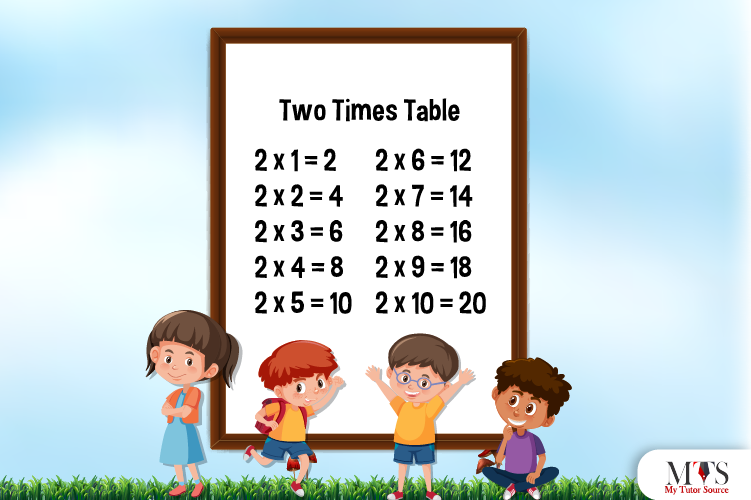# Multiplication Table of 2 – Tips to Memorize 2 Times Table & Example QuestionsTable of 2 is the first step to making mental arithmetic easier. Multiplication skills are crucial for understanding intimidating and complex problems of mathematics. Math tutors and professors teach multiplication tables 1 to 20 times in different formats. Math comes with tips and tricks – that’s something quite interesting about it.

Let us help you memorize multiplication tables up to 20 to save your time during the competitive examination. This blog is about the table of 2 with different formats, learning tips, and a few solved examples.

## Table of 2

The multiplication table of 2 includes the product or multiplies of the number 2. It represents the addition of 2 digits when multiplied by any other whole number or consecutive positive integers. Whether it’s a school teacher or private math tutor of junior classes, they help students memorize a table of 2 by heart. As it promotes a better understanding of numbers and their relationships. Other than this, table of 2 is famous for forming the base of multiplication problems. Here we go with the table of 2 up to 20 times. Read it out:

2 x 1 = 2
2 x 2 = 4
2 x 3 = 6
2 x 4 = 8
2 x 5 = 10
2 x 6 = 12
2 x 7 = 14
2 x 8 = 16
2 x 9 = 18
2 x 10 = 20
2 x 11 = 22
2 x 12 = 24
2 x 13 = 26
2 x 14 = 28
2 x 15 = 30
2 x 16 = 32
2 x 17 = 34
2 x 18 = 36
2 x 19 = 38
2 x 20 = 40

## Method to Read Table of 2

This is how we read the multiplication table of 2 for learning purposes:

One time two is two (2)
Two times two is four (4)
Three times two is six (6)
Four times two is eight (8)
Five times two is ten (10)
Six times two is twelve (12)
Seven times two is fourteen (14)
Eight times two is sixteen (16)
Nine times two is eighteen (18)
Ten times two is twenty (20)
Eleven times two is twenty-two (22)
Twelve times two is twenty-four (24)
Thirteen times two is twenty-six (26)
Fourteen times two is twenty-eight (28)
Fifteen times two is thirty (30)
Sixteen times two is thirty-two (32)
Two times two is thirty-four (34)
Eighteen times two is thirty-six (36)
Nineteen times two is thirty-eight (38)
Twenty times two is forty (40)

## Tips for Memorizing Multiplication Table of 2

Obtaining a table of 2 is relatively easy, even for kids. Here are a few tips that will help you memorize the table of 2. Choose a tip that matches your learning style, or you find easier than others. You just have to observe a few basic things in the 2 times table, such as:

### Tip # 1:

Multiplication table of 2 is formed by the set of even numbers, i.e., 2, 4, 6, 8, 10, 12, 14, 16, 18, 20 …

### Tip # 2:

Good at counting? Skip count by 2, and you will get the 2 times table.

### Tip # 3:

Memorize table of 2 by using the addition. Add 2 to the multiple of the table of 2 to get the next multiple. We have designed the following table to help you practice this tip:

## Example Question of Table of 2

One of the great teaching strategies followed by private math tutors to help students memorize the table of 2 is letting them solve short questions. Here are a few examples of questions related to the multiplication table of 2. Kids must practice them all for fast and long-term learning.

Question: What is equal to 2 times 9?

According to table of 2,
2 times 9 = 2 x 9 = 18

Question: Find 2 times 2 times 2 by using the multiplication table of 2?

Given that,
2 times 2 times 2
In mathematical form, it is written as
2 x 2 x 2
Using 2 times table, we get
2 x 2 x 2 = 8
Hence, 2 times 2 times 2 is 8

Question: What is 2 times 5 plus 2 times 4?

2 times 5 = 2 x 5 = 10
2 times 4 = 2 x 4 = 8
Therefore,
2 times 5 plus 2 times 4 = 2 x 5 + 2 x 4 = 10 + 8 = 18

Question: If Leena buys 2 chocolates per day, using the table of 2 find out how many chocolates she buys within 9 days?

Chocolates per day = 2
Chocolates within 9 days = 2 x 9 = 18
Hence, Leena buys 18 chocolates within 9 days.

Question: 2 multiplied by what number results in 48?

Let ‘Q’ be the number
In mathematical form
2 x Q = 48
Q = 48/2
Q = 24
Hence, 2 multiplied by 24 results in 48

### Find Top Tutors in Your AreaWith over 3 years of experience in teaching, Chloe is very deeply connected with the topics that talk about the educational and general aspects of a student's life. Her writing has been very helpful for students to gain a better understanding of their academics and personal well-being. I’m also open to any suggestions that you might have! Please reach out to me at chloedaniel402 [at] gmail.com